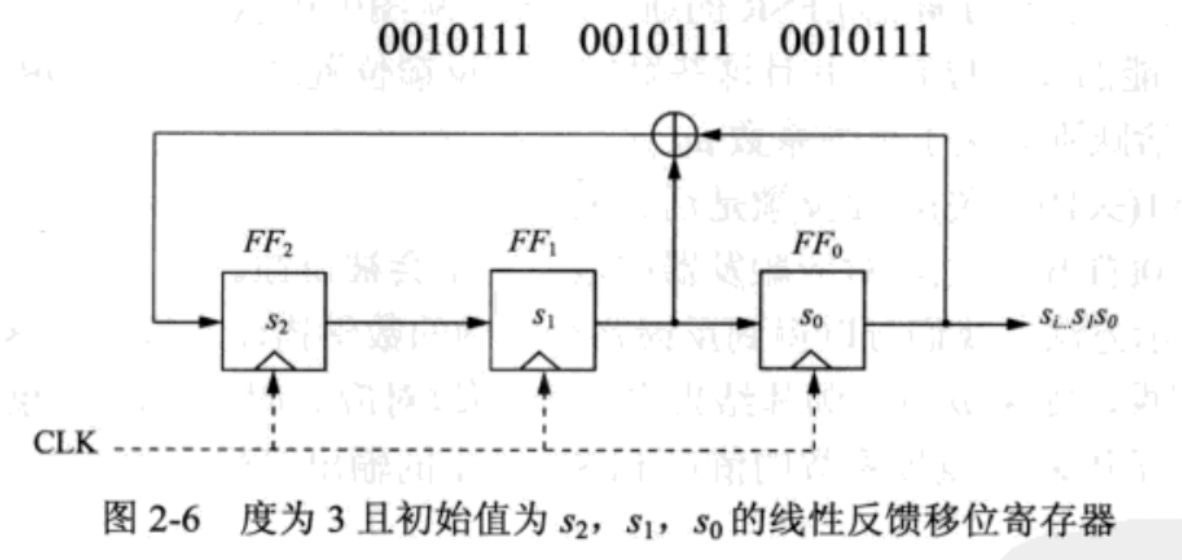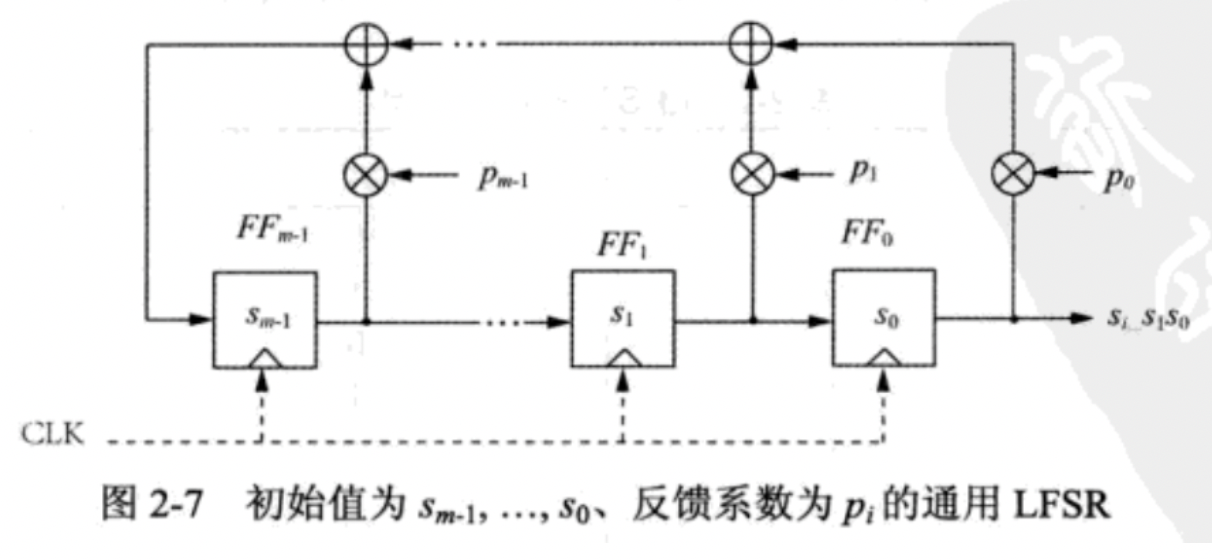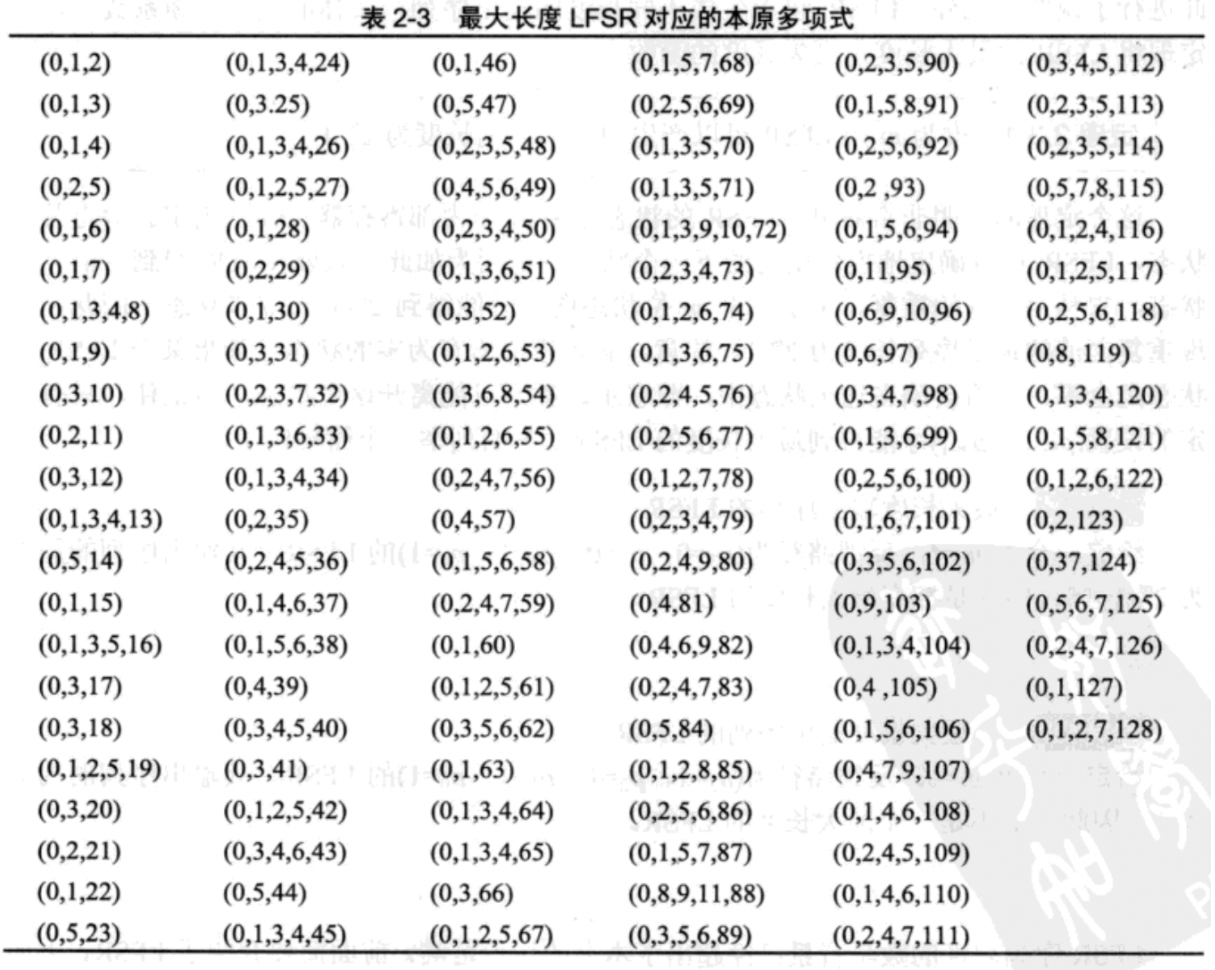0%

# LFSR

• LFSR作用

产生长伪随机序列，通常为二进制

• 存储元件（触发器）

存储数据的一个概念，知道可以存数据即可。

• 反馈路径

计算移位寄存器中某些触发器的XOR和，并将其传递。

• LFSR由若干时钟存储元件（触发器）组成，而存储元件的个数就是LFSR的度。

## 简单LFSR1. 假设初始状态为：S2=1, S1=0, S0=0 ；最右位的FF0往外输出

2. 在每一个时间单位下，内部状态位向右移动一位

从左往右看

• 原先S2的值（1）给到 FF1
• 原先S1的值（0）给到 FF0
• 原先S0的值（0）和 原先S1的值（0）异或后（得到0）给到 FF2
• 原先S0的值（0）输出，LFSR得到数据 0
3. 多次移位后，得到数据

Clk FF2 FF1 FF0=si
0 1 0 0
1 0 1 0
2 1 0 1
3 1 1 0
4 1 1 1
5 0 1 1
6 0 0 1
7 1 0 0
8 0 1 0

$$S3 ≡ S1 + S0 \mod 2$$

$$S4 ≡ S2 + S1 \mod 2$$

$$S5 ≡ S3 + S2 \mod 2$$

$$…$$

$$S_{i+3} ≡ S_{i+1} + S_i \mod 2$$

## LFSR通用形式

• 反馈系数

某条反馈路径是否活跃取决于反馈系数P0, P1, …, Pm-1

• 若Pi = 1，此反馈是活跃的
• 若Pi = 0，对应触发器的输出将不会被反馈1. 它的值给到下一个触发器
2. 还有条支路，导致 Sm-1 需要去乘 Pm-1，再异或

$$S_m ≡ S_{m-1}P_{m-1} +···+ S_1P_1 + S_0P_0 \mod 2$$

$$S_{m+1} ≡ S_{m}P_{m-1} +···+ S_2P_1 + S_1P_0 \mod 2$$

$$S_{i+m} ≡ \sum_{j=0}^{m-1} P_{j}·S_{i+j} \mod 2; S_i, P_j∈{0, 1}; i = 0, 1, 2, …$$

• LFSR的输出由m个内部寄存器状态唯一确定

• 给定某个状态，也能确定下一个状态

### 本原多项式

LFSR还可以用多项式表达

$$P(x)=x^m+P_{m-1}x^{m-1}+…+P_1x+P_0$$### LFSR · 已知明文攻击

• 度： m

• 已知明文： x0, x1, …, x2m-1

• 对应密文： y0, y1, …, y2m-1

$$S_i ≡ x_i + y_i \mod 2; i=0, 1, …, 2m-1$$

$$S_{i+m} ≡ \sum_{j=0}^{m-1} P_{j}·S_{i+j} \mod 2; S_i, P_j∈{0, 1}; i = 0, 1, 2, …$$

$$i=0, S_m ≡ P_{m-1}S_{m-1}+…+P_1S_1+P_0S_0 \mod 2$$

$$i=0, S_{m+1} ≡ P_{m-1}S_{m}+…+P_1S_2+P_0S_1 \mod 2$$

$$…$$

$$i=m-1, S_{2m-1} ≡ P_{m-1}S_{2m-2}+…+P_1S_m+P_0S_{m-1} \mod 2$$